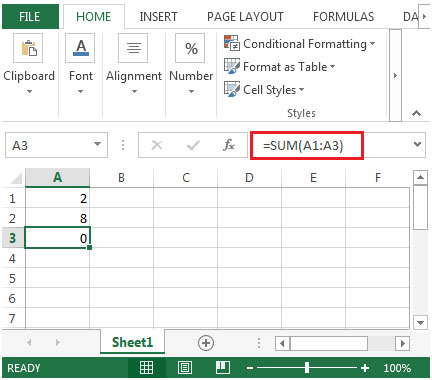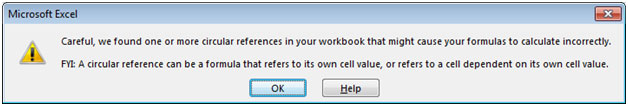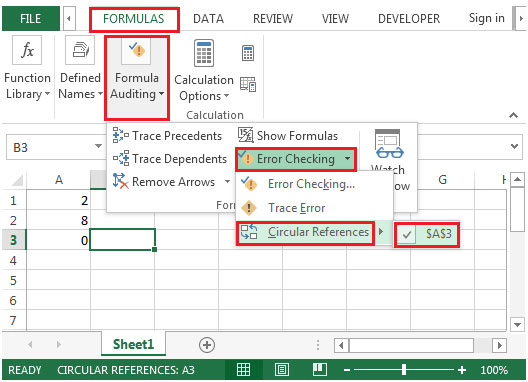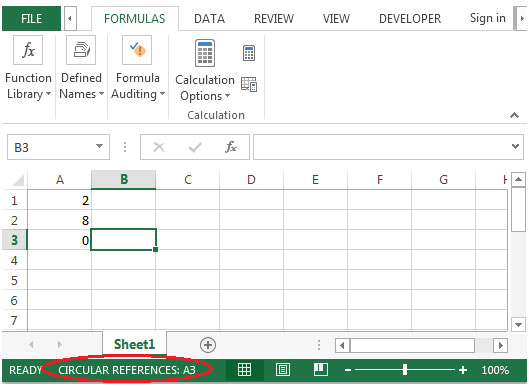# Circular Reference in Microsoft Excel

Circular reference can be a formula that refers to its own cell value, or refers to a cell dependent on its own cell value.

In simple words, the cell in which formula is being calculated & the cell itself is also included or part of the range then a circular reference warning message will appear. It might cause for the incorrect calculation by the formulas.

Let us take an example:

• In cell A1 & A2, numbers are entered & in cell A3 we want to find out the total.• While typing the sum formula, the range is stretched to cell A3 in which formula is entered.
• Excel will pop-up an error messageIf above shown error message regarding circular reference pops up while adding formulas, then you might have created circular cell reference unknowingly. You can track & then remove the incorrect reference & it will remove the circular reference.

To track circular reference, follow the below steps:

• Click on blank cell
• Click on Formulas ribbon
• In Formula Auditing group, click on Error Checking
• Select Circular References & it will provide the location of the cell in which circular reference occurs.• If there are multiple circular references, then you are required to manually go to each cell & correct the formula until the status bar no longer shows the word “CIRCULAR REFERENCES”Below you can find more examples:-